American Journal of Theoretical and Applied Statistics
Volume 6, Issue 1, January 2017, Pages: 44-51

Multivariate Regression Analysis of Oil Price Volatility on GDP Growth in Kenya

Anthony Makau

Macroeconomic Statistics, Kenya National Bureau of Statistics, Nairobi, Kenya

Email address: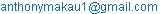To cite this article:

Anthony Makau. Multivariate Regression Analysis of Oil Price Volatility on GDP Growth in Kenya. American Journal of Theoretical and Applied Statistics. Vol. 6, No. 1, 2017, pp. 44-51. doi: 10.11648/j.ajtas.20170601.16

Received: January 6, 2017; Accepted: January 16, 2017; Published: February 20, 2017

Abstract: Despite Oil being one of the key drivers of the world economy, the recent fluctuations in oil prices has brought concerns about possible slowdowns in economic growth globally. To cushion their economies from these oil price volatility shocks, a number of developing countries have made structural reforms in their macroeconomic policies as far as domestic petroleum pricing system is concerned. In line with this, Kenya has undertaken to reform the energy sector so as to make it competitive, efficient as well as attracting investment in the sector. The main objective of this study was to investigate if volatility of oil price had an effect on Kenya’s GDP growth rate with Exchange rate and Inflation rate as intervening variables. The study used quarterly data from KNBS, CBK and ERC for the periods 2004 to 2013 to achieve its objective and all analysis were done in R. Analysis showed that fluctuation of Crude oil price in the international market coupled with fluctuations in the exchange rate and inflation rate determined 86.9 per cent of the trend in GDP growth rate. The study found that when crude oil price increases by KSh 1,000 per barrel, the Kenya shilling weakens by a single Kenya shilling for every US dollar and the inflation rate goes up by 1 per cent, then the GDP growth rate decreases by 0.132 percentage points (p=0.000). The study also found that the model used had no serial autocorrelation meaning that the error terms of the regression model at any given two different quarters were linearly uncorrelated. Moreover, Goldfeld-Quandt test statistic was found to be significantly higher than 5% or 1% significance levels. This was despite a plot graph of residuals vs the fitted values of GDP growth rate showing unequal distribution of residuals as the values of fitted GDP growth rate increased. Therefore the model was free from heteroscedasticity. The government should therefore focus on stabilizing exchange rate, increase domestic energy production to reduce reliance on importation of petroleum products and control the level of inflation.

Keywords: Ordinary Least Square, Balance of Payments, Best Linear Unbiased Estimator, Foreign Direct Investment, Heteroscedasticity, Serial Autocorrelation

1. Introduction and Literature Review

1.1. Background of the Study

Oil is one of the key drivers of the economy others being economic output, unemployment, inflation, savings and investments. Since its discovery in the 20thcentury, demand of oil and oil products mostly used in industries and automobiles has been constantly growing. Fluctuations of international oil price in the recent years have proven to be sources of vulnerability to developing economies. Just like any other developing countries, Kenya has had a lot of setback in its economic performance; from the ever rising inflation, fluctuation in exchange rates among others. Oil prices have acted as a major economic burden since global oil pricing of this crucial commodity is determined entirely by oil exporting countries, such as Saudi Arabia which produces 40% of the global oil and has 73% of the world’s proven oil reserves. This is reflected in the country’s current account of the Balance of Payment (BOP) statistics which has been worsening following the escalating international oil prices due to high demand for oil which has ballooned import bill, coupled with the weakening of the Kenya shilling against major world currencies such as the US dollar.

1.2. Review of Previous Studies on the Subject of Study

Jimenez-Rodriguez & Sanchez (2005) examined the effects of oil price shocks on the real economic activity of the major industrialized countries. They concluded that, oil price increase have an impact on GDP growth of a large magnitude than that of oil price declines, with the latter being statistically insignificant in most cases. Further, among the oil importing countries, oil price increase were found to have a negative impact on the economic activities . A study on the impact of oil price shock and exchange rate volatility on economic growth conducted by Jin (2008) in Japan, the second largest net importer of crude oil after the United States, revealed that oil price increases exert negative impact on economic growth of both countries. Jin (2008) further accredited that the real GDP growth of Japan dropped from 2.5 per cent in 2006 to 1.6 per cent in 2007 owing to oil price shocks . According to Mecheo and Omiti’s study of 2003, petroleum is a major source of energy in Kenya and accounts for over 80 per cent of the country’s commercial energy requirement. However, the study noted that changes in the international oil price are the reason behind the fluctuating import bill on petroleum imports . Li and Zhao (2011) observed that crude oil price fluctuations from 1970s to 2011 have been increasingly erratic. This has led to worsening of terms of trade and BOP’s current account of oil importing countries like Kenya with an adverse impact on businesses, consumers, government budget and the economy at large . Increase in energy prices lead to a considerable rise in production and transportation costs and as a result, wages and inflation goes up, leading to stunted economic growth (O’Neill, Penm and Terrell, 2008) . Oriakhi and Osaze (2013) established that oil price volatility had a direct impact on real government expenditure, real exchange rate and real import, real money supply and inflation . The relationship between crude oil price and economic growth varies depending on a country’s sectoral composition, institutional structures and macroeconomic policies among others (Chuku et al 2010) .

1.3. Statement of the Problem

Gonzalez and Nabiyev study in 2009 points out that fluctuation of oil price which have become more pronounced than they were in the 1990s have led to unpredictable consequences in an economy . To be able to draw macroeconomic policies in a bid to cushion the economy from these oil price volatility shocks, it is necessary to establish the relationship between the country’s macroeconomic indicators and petroleum oil price fluctuations.

1.4. Significance of the Study

The study would be an eye opener to the current and would be investors in Kenya as they seek to know the vulnerability of investing in the economy. This is a key decision factor especially on Foreign Direct Investment. The government will be able to make informed policies that guide petroleum importation as well as other pertinent substitutes such as hydroelectric and renewable sources of energy to mitigate reliance on a single and unstable source of energy.

1.5. Objectives

General

Investigate the effect of oil price volatility on Kenya’s GDP growth rate using Multivariate Regression technique, exchange rate and inflation rate being the intervening variables.

Specific

i.   Derive Multivariate parameter estimates using Ordinary Least Squares (OLS) method.

ii.   Validate the OLS parameter estimates by testing and correcting for serial autocorrelation and heteroscedasticity.

iii.   Ascertain oil price volatility effect on Kenya’s GDP growth rate, with exchange rate and inflation rate as intervening variables.

1.6. Study Limitations

The findings of this study are limited to the years 2004 to 2013. Thus the finding is a statistic and not a population parameter which is subject to an error margin. The exchange rate regime has undergone through numerous regimes making the study unrepresentative of the previous regimes. In this study, the exchange rate used was for US dollar to Kenya shillings. This is because most of Kenya’s imports especially petroleum products are bought using the US dollar.

2. Methodology

2.1. Introduction

This chapter discusses the methodology used to achieve the objectives under study.

2.2. Data Collection Technique

Data used for this study was sourced from administrative records. This included international crude oil prices from ERC, exchange rate (US Dollars to KSh) from CBK and GDP growth rate; and Inflation rate from KNBS.

2.3. Multivariate Model

The study examined if the trend in GDP growth rate can be explained by fluctuations of oil prices, exchange rate and inflation rate using multivariate linear regression model. Ordinary least square method was used to get the parameter estimates of the model. Multivariate model used to determine the effect of crude oil price on GDP growth rate as intervening variables were introduced one at a time was as follows;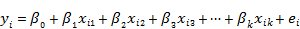(1)

2.4. Ordinary Least Square Parameter Estimation Method

Considering the above model,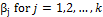is the measure of change in the dependent variable y corresponding to a unit change in the independent variable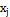with the other independent variables remaining constant. From equation (1) it follows that the OLS can be obtained by;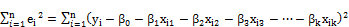(2)

The above OLS can be minimized by differentiating partially equation (2) with respect to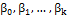respectively and equating them to zero and replace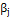with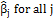. From this procedure, (k+1) normal equations are obtained as follows;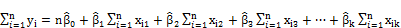(3)

Multiplying the above equation by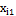and similarly repeating this procedure by multiplying equation by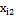then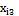until. The equations obtained are (k+1) normal equations;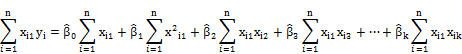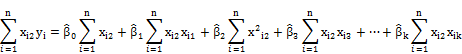.

.

.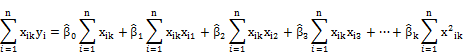These k+1 normal equations can be re-written in matrix notation as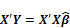(4)

Where: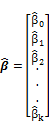,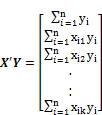and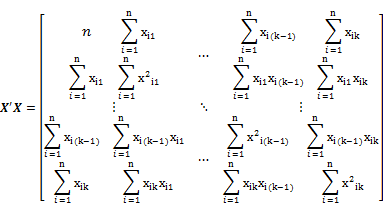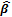is a column vector of OLS parameter estimates and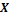is of full rank and the inverse of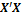exists , .

Thus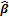, which is the OLS parameter estimate of β becomes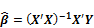(5)

2.5. Diagnostic Tests

2.5.1. Serial Autocorrelation

When the error term in one time period is positively correlated with the error term in the previous time period, we have the 1st order positive autocorrelation.

Consider this model for illustration;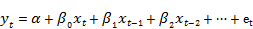(6)

Where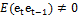i.e. they are correlated

Let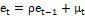Where;is some constant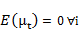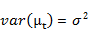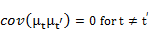Test for autocorrelation (Durbin Watson test)

The presence of 1st order autocorrelation is detected by testing the significance ofinin the following hypothesis;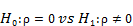At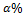level of significance.

Durbin and Watson devised a statistic to test the above hypothesis. The test statistic is defined as;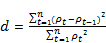(7)

Where;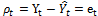and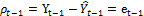The above test statistic satisfies the inequality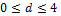and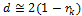, where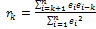is the kth order serial correlation. If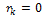then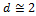, thus we fail to reject the null hypothesis implying that there is no serial correlation at the kth order. Values of d close to 0 indicate positive serial autocorrelation, while values of d close to 4 imply negative serial autocorrelation .

2.5.2. Heteroscedasticity

If the OLS assumption that the variance of the error term is constant for all values does not hold, then we have the problem of heteroscedasticity.

Consider the model,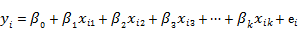Where:

i.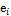is normally distributed

ii.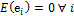iii.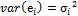iv.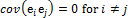With heteroscedasticity, the OLS estimates are still unbiased and consistent but inefficient (not BLUE) , .

Test for heteroscedasticity (Goldfelt-Quandt test)

The presence of heteroscedasticity in a two variable linear model can be tested by performing 2 separate regressions;

i      For the small values of independent variable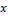and

ii     For large values of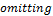about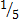of the total number of observations lying in the middle.

The ratio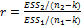is tested to see if it’s significantly different fromusing the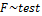Where;

a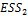is the Error Sum of Squares of the 2ndregression

b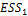is the Error Sum of Squares of the 1stregression

c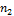and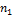are the number of observations in the 2ndand 1st regression

d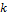is the number of estimated parameters

The hypothesis is stated as;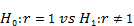The criterion will be to reject the null hypothesis of homoscedasticity if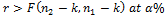level of significance. If the hypothesis is not rejected, it implies that our model has unequal variance in the error term which can be corrected by transforming the linear model to obtain a homoscedasticity model; provided the assumption that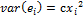holds, and consequently the OLS parameter estimates obtained using the new model will be a Best Linear Unbiased Estimator (BLUE) of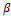.

Correcting for heteroscedasticity

The study will achieve this by transforming the above linear model by dividing it by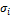to obtain a model which is free from heteroscedasticity.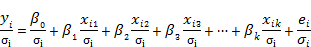Which we can denote as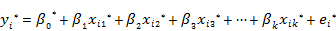(8)

Where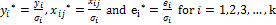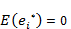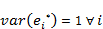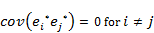Where equation (8) is the new linear model free from heteroscedasticity i.e. homoscedastic model , .

3. Results and Discussion

3.1. Preliminary Analysis

GDP growth rate had a negative correlation with each of the three explanatory variables. As presented in Table1, every time crude oil price goes up by KSh 1,000, GDP growth rate dips by 0.165 percentage points. Similarly, if the Kenya shilling weakens against the US dollar by a single shilling and inflation rate goes up by 1 per cent, GDP growth rate decelerates by 0.059 and 0.470 percentage points respectively. If crude oil price goes up inflation also increases, if the shilling weakens against the US dollar, crude oil price goes up and finally if the shilling weakens against the US dollar the inflation goes up.

Table 1. Correlation coefficient matrix of the variables.

 GDP growth rate Crude oil price Exchange rate Inflation rate GDP growth rate 1.0000 Crude oil price -0.1646 1.0000 Exchange rate -0.0591 0.5878 1.0000 Inflation rate -0.4700 0.0992 0.1877 1.0000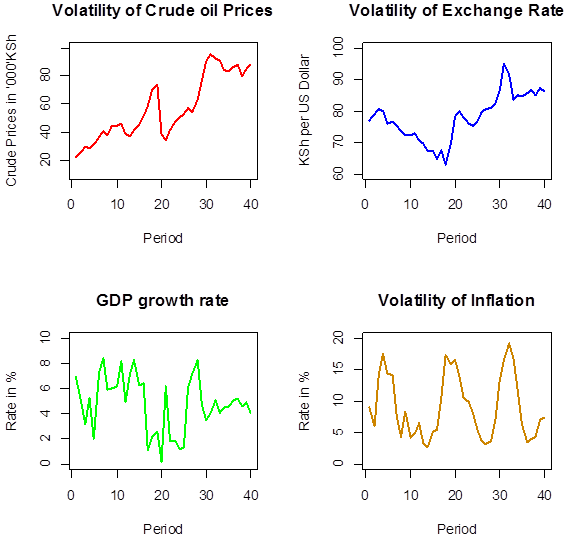Figure 1. Crude oil price, Exchange rate, Inflation and GDP growth rate trends for 2004 to 2013.

3.2. Regression Analysis

3.2.1. Fitting Multivariate Regression Model

The fitted multivariate regression model was as follows;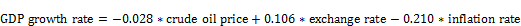(9)

Interpretation of the fitted model

When crude oil price increase by KSh 1,000 per barrel, the Kenya shilling weakens by a single Kenya shilling for every US dollar and the inflation rate goes up by 1 per cent, the GDP growth rate decreases by 0.132 percentage points (p=0.000). However, this decrease in GDP growth rate is 86.9 per cent of the actual decline as the model assumes in the absence of other indicators such as interest rates, the remaining 13.1 per cent is due to stochastic nature of the model.

3.2.2. Effect of Intervening Variables to the Multivariate Regression Model

Intervening variables were introduced to the regression model one at a time and the regression coefficient of the models tabulated in Table 2.

Table 2. Regression coefficients of model with various Intervening variables introduced.

 Model Variables R2 Adjusted R2 P-value Model1 No intervening variable 0.6816 0.6735 3.082e-11 Model2 Inflation rate as the only intervening variable 0.6829 0.6662 3.328e-10 Model3 Exchange rate as the only intervening variable 0.8315 0.8226 2.019e-15 Model4 Both Exchange and Inflation rates as intervening variables 0.8691 0.8585 <2.2e-16

Analysis shows that fluctuation of crude oil price could only account for 68.2 per cent of the trend in GDP growth without any intervening variable being introduced in the model. Exchange rate was the most significant intervening variable to introduce to the model as compared to inflation rate. Regression coefficient shows that fluctuations in both the crude oil price and exchange rate explains 83.2 per cent of the behavior in GDP growth rate, while introducing the Inflation rate the adjusted R2 goes down by 1.3 percentage points meaning introducing inflation rate alone to the model was insignificant in explaining the trend in GDP growth rate. However 86.9 per cent of the trend in GDP growth rate was explained by fluctuation of crude oil price coupled with fluctuations in exchange rate and inflation rate.

3.3. Validating the Ordinary Least Square Parameter Estimates

In order to validate the OLS parameter estimates, a series of diagnostic tests were done.

3.3.1. Durbin-Watson Test Statistics for Serial Autocorrelation

Hypothesis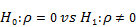Test statistics

Durbin Watson statistic=1.5034

Interpretation and Conclusion

Since 1.5034 is not significantly different from 2 than it is to either 0 or 4; we fail to reject the null hypothesis at 5% significance level. The model therefore had no serial autocorrelation implying that the error terms of the regression model for any given two different quarters were linearly uncorrelated.

3.3.2. Test Statistics for Heteroscedasticity

A plot of residuals vs the fitted values of GDP growth rates was done to study the presence of heteroscedasticity.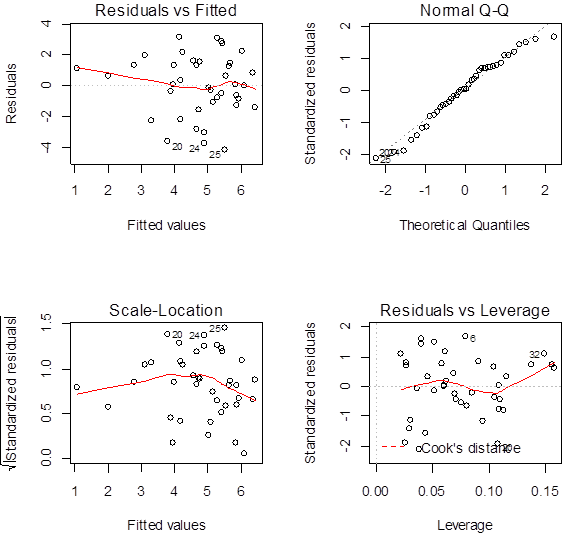Figure 2. Plot of the relation of residuals and fitted values of GDP growth rates.

In Figure 2, the top-left plot graph of residuals vs the fitted values of GDP growth rate, the graph shows that the residuals are not equally distributed as they seem to increase as the fitted GDP growth rate values increase implying the presence of heteroscedasticity.

Goldfeld-Quandt test statistic

HypothesisTest statistics

Goldfeld-Quandt statistic=0.90611, df1=17, df2=17, p-value=0.5794

Interpretation and Conclusion

Since the calculated p-value for the Goldfeld-Quandt test is significantly higher than 5% or 1% significance levels, the null hypothesis of homoscedasticity is not rejected. This is despite graph of residuals vs the fitted values of GDP growth rate showing unequal distribution of residuals as the values of fitted GDP growth rate increased. Therefore traces of heteroscedasticity exist although very insignificant at the given significance levels. The Multivariate Regression linear model was therefore found to be homoscedastic i.e. free from heteroscedasticity.

4. Conclusions and Recommendations

The Government should focus on stabilizing the exchange rate. A stable exchange rate will prevent significant fluctuation of the oil import bill attributed to the unexpected changes in the exchange rate. Secondly, the Government should increase domestic energy production in order to reduce its reliance on imported oil. This could be achieved through increasing the production of cheap and reliable energy such as solar, wind, coal and geothermal energy. In addition, the recently discovered oil wells should be exploited to meet the country’s oil demand. This is likely to reduce oil imports, there by promoting economic growth through a stable supply of cheap energy. Finally, controlling the level of inflation is very key for a sustainable economic growth. Therefore, policymakers should put measures in place that would keep inflation rate at low level.

Acknowledgement

My acknowledgement goes to Dr. Waititu and Dr. Mung’atu of Jomo Kenyatta University of Agriculture and Technology (JKUAT) for the knowledge they have imparted on me. Mr. Etwasi and Ms. Mercylline for their moral support.

R-CODE

 a1<-cor(GDP.growth,GDP.growth) a2<-cor(GDP.growth,Crude.Oil.Price) a3<-cor(GDP.growth,Exchange.Rate) a4<-cor(GDP.growth,Inflation.Rate) b1<-cor(Crude.Oil.Price,Crude.Oil.Price) b2<-cor(Crude.Oil.Price,Exchange.Rate) b3<-cor(Crude.Oil.Price,Inflation.Rate) c1<-cor(Exchange.Rate,Exchange.Rate) c2<-cor(Exchange.Rate,Inflation.Rate) d1<-cor(Inflation.Rate,Inflation.Rate) #Correlationmatrix m<-matrix(c(a1,a2,a3,a4,a2,b1,b2,b3,a3,b2,c1,c2,a4,b3,c2,d1),nrow=4,ncol=4,byrow=TRUE) m # par(mfrow=c(2,2)) plot(Crude.Oil.Price,type="l",lwd=2,col="red",main="VolatilityofCrudeoilPrices",xlab="Period",ylab="CrudePricesin'000'KSh",ylim=c(10,100)) plot(Exchange.Rate,type="l",lwd=2,col="blue",main="VolatilityofExchangeRate",xlab="Period",ylab="KShperUSDollar",ylim=c(60,100)) plot(GDP.growth,type="l",lwd=2,col="green",main="GDPgrowthrate",xlab="Period",ylab="Ratein%",ylim=c(0,10)) plot(Inflation.Rate,type="l",lwd=2,col="orange3",main="VolatilityofInflation",xlab="Period",ylab="Ratein%",ylim=c(0,20)) # #withoutinterveningvariables model1<-lm(GDP.growth~-1+Crude.Oil.Price) summary(model1) #withexchangerateasinterveningvariable model2<-lm(GDP.growth~-1+Crude.Oil.Price+Exchange.Rate) summary(model2) #withinflationrateasinterveningvariable model3<-lm(GDP.growth~-1+Crude.Oil.Price+Inflation.Rate) summary(model3) #withExchangeandInflationratesasinterveningvariables model4<-lm(GDP.growth~-1+Crude.Oil.Price+Exchange.Rate+Inflation.Rate) summary(model4)# #SerialCorrelation #Durbin&WatsontestforSerialCorrelation library(lmtest) dwtest(model4) # #Heteroscedasticity #GoldfeldQuandttestforHeteroscedasticity gqtest(model4) #plottingtheresidualsversusthepredictedvalues par(mfrow=c(2,2)) plot(model4) #

References

1. Jiménez-Rodríguez, R., & Sánchez, M. (2005). Oil price shocks and real GDP growth: empirical evidence for some OECD countries. Applied economics, 37 (2), 201-228.
2. Jin, G. (2008). The Impact of Oil Price Shock and Exchange Rate Volatility on Economic Growth: A comparative analysis for Russia, Japan, and China. Research Journal of International Studies, 8 (11), 98-111.
3. Mecheo, J., & Omiti, J. (2003). Petroleum market structure and pricing following deregulation. Institute of Policy Analysis and Research.
4. Li, Z., & Zhao, H. (2011). Not all demand oil shocks are alike: disentangling demand oil shocks in the crude oil market. Journal of Chinese Economic and Foreign Trade Studies, 4 (1), 28-44.
5. O'Neill, T. J., Penm, J., & Terrell, R. D. (2008). The role of higher oil prices: a case of major developed countries. Research in Finance, 24, 287-299.
6. Oriakhi, D. E., & Osaze, I.D. (2013). Oil Price Volatility and Its Consequences on the Growth of the Nigerian Economy: An Examination (1970-2010). Asian Economic and Financial Review, 3 (5), 683.
7. Chuku, C. A., Effiong, E. L., & Sam, N. R. (2010). Oil price distortions and their short-and-long-run impacts on the Nigerian economy. In Proceedings of the 51st Annual Conference of the Nigerian Economic Society.
8. Gonzalez, A., & Nabiyev, S. (2009). Oil price fluctuations and its effect on GDP growth: A case study of USA and Sweden. Jonkoping International Business School.
9. Pindyck, R. S. & Rubinfeld, D. L. (1998). Econometric Models and Economic Forecasts, 4thEdition, Irwin/McGraw-Hill.
10. Gujarati, D. N. (2004). Basic Econometrics, 4thEdition, the McGraw−Hill Companies.
11. Goldfeld, S. M. & Quandt, R. E. (1965). Some Tests for Homoskedasticity. Journal of the American Statistical Association. 60, 539–547.

 Contents 1. 1.1. 1.2. 1.3. 1.4. 1.5. 1.6. 2. 2.1. 2.2. 2.3. 2.4. 2.5. 3. 3.1. 3.2. 3.3. 4.
Article ToolsAbstractPDF(249K)
Follow on us
PUBLICATION SERVICE
JOIN US
RESOURCES
SPECIAL SERVICES
ADDRESS
Science Publishing Group
548 FASHION AVENUE
NEW YORK, NY 10018
U.S.A.
Tel: (001)347-688-8931